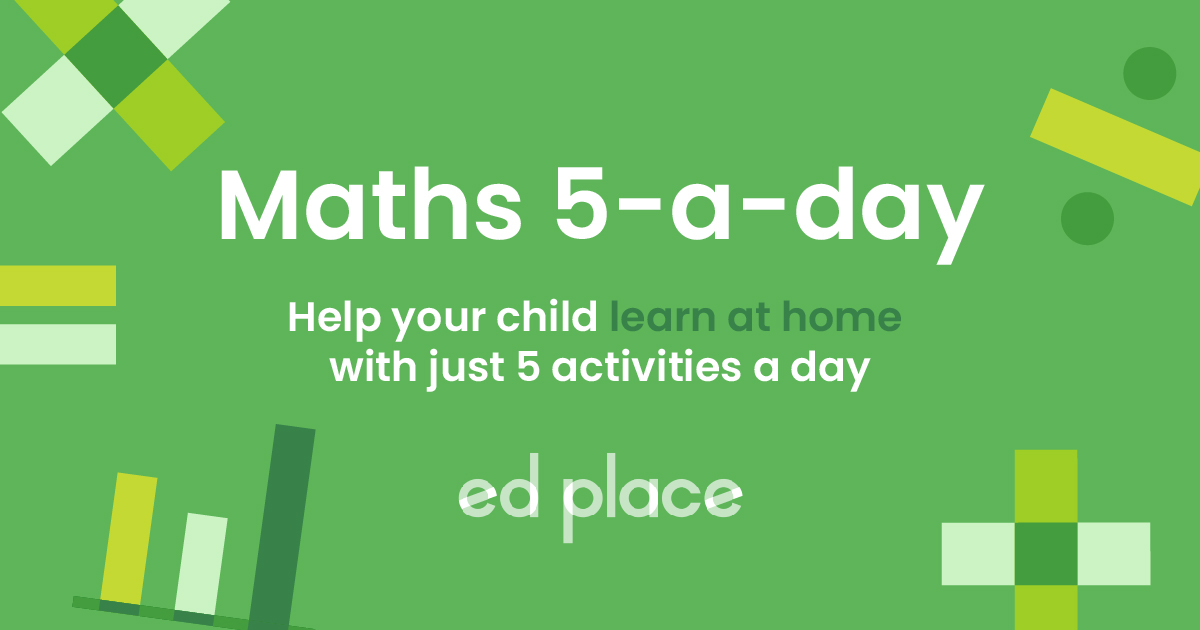# EdPlace's Upper KS2 home learning maths lesson: Long Division

Looking for short lessons to keep your child engaged and learning? Our experienced team of teachers have created English, maths and science lessons for the home, so your child can learn no matter where they are. Plus, as all activities are automatically marked, you really can encourage your child to be an independent learner.

Get them started on the lesson below and then jump into our teacher-created activities to practice what they've learnt. We've recommended five to ensure they feel secure in their knowledge - 5-a-day helps keeps the learning loss at bay (or so we think!).

Are they keen to start practising straight away? Head to the bottom of the page to find the activities.

Now...onto the lesson!

Key Stage 2 statutory requirements for maths
Year 5  students should be able to divide numbers up to 4 digits by a one-digit number using the formal written method of short division
Year 6  students should be able to divide numbers up to 4 digits by a two-digit whole number using the formal written method of long division

# Does the Bus Stop Method Cause a Gridlock in Your House?

How long is it since you had to use long division?  It's a topic that often causes a bit of worry for both students and parents alike.  However, although it seems like a daunting Maths topic, once learnt the correct methods it’s really quite straightforward.  We're going to look at how to divide 4-digit numbers by 2-digit numbers, using the method of long division.
It’s a good one to keep practising every now and then, as it's easy to forget and can be incredibly handy at times!

We believe that if you follow our step by step approach your child will:

1) Understand how to divide any whole 4-digit number by a whole 2-digit number

2) Apply this to independent work

3) Explain to you how they completed their work

## Step 1 - Division Definitions

The dividend is the number you are dividing by – the larger number.
The divisor is the number you are dividing into that larger number – the smaller number.
The ‘bus stop method’ is the way we lay out the question – it looks like a bus stop! See Step 2 for an example.
The remainder is the number left over if a number doesn’t multiply exactly into another.

## Step 2 - Mastering key concepts first...

Your child will need to have an understanding of the bus stop method and how to divide a one-digit number into a larger number:
654 ÷ 3 = 218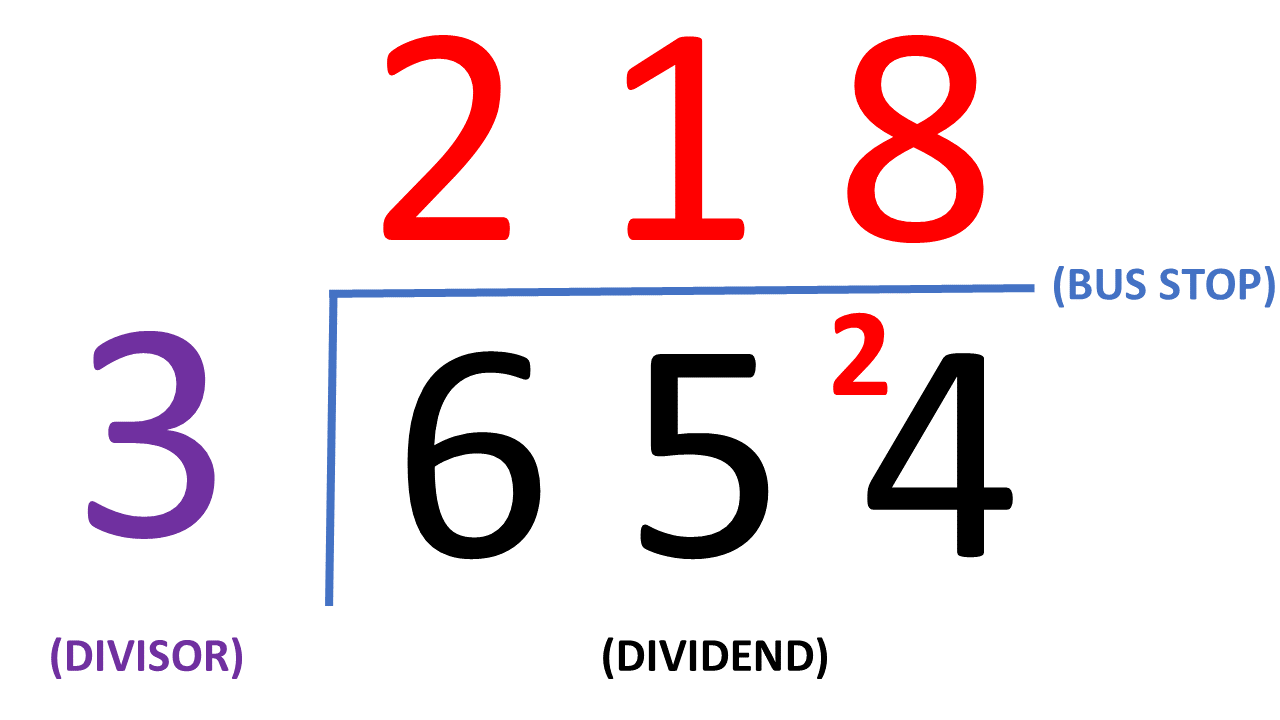How many 3's go into 6? ... 2

How many 3's go into 5? ... 1 with a remainder 2

How many 3's go into 24? ... 8

## Step 3 – Long Division with Larger Numbers

Long division is basically the same as we've just seen in Step 2, but with larger numbers!

4,811 ÷ 17 = ?

The very first thing you must always do is write out the multiples of the divisor (in this case, 17). This can be tricky when working with larger numbers – you just need to keep adding the number on and on, until you have ten multiples.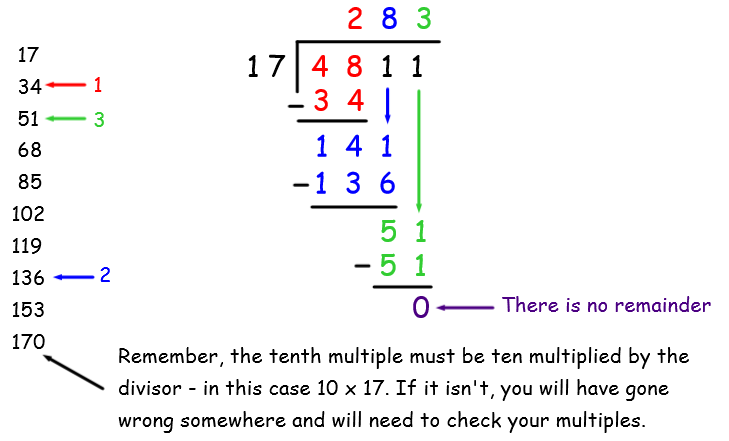Write out the multiples of 17 – check the tenth multiple is 10 x 17
How many 17s go into 4? ... 0.  So, how many 17s go into 48?... 2 (write 2 at the top)
2 x 17 = 34, so we put the 34 underneath 48 so we can work out the remainder (by subtracting)
The remainder is 14. Instead of writing it up next to the 1, we drop the 1 down instead because there’s more space!
How many 17s go into 141? ... 8 (write 8 at the top)
8 x 17 = 136, so we put 136 underneath 141 to work out the remainder (subtract)
The remainder is 5. We drop the next digit from the dividend down and 5 becomes 51.
How many 17s go into 51?... 3 exactly, so we put the 3 up in our answer and there is no remainder.

4,811 ÷ 17 = 283

## Step 4 – Long Division with Larger Numbers

Now have a go at these examples together:
9,129 ÷ 17
9861 ÷ 19

Challenge questions:
4,761 ÷ 23
3,988 ÷ 51

## Step 5 – Get Practising

Now that you’ve learnt long division together, see if your child can apply their learning to the following 5 activities. Have them complete them in this order:

All activities are created by teachers and automatically marked. Plus, with an EdPlace subscription, we can automatically progress your child at a level that's right for them. Sending you progress reports along the way so you can track and measure progress, together - brilliant!

a) 537
b) 519
c) 207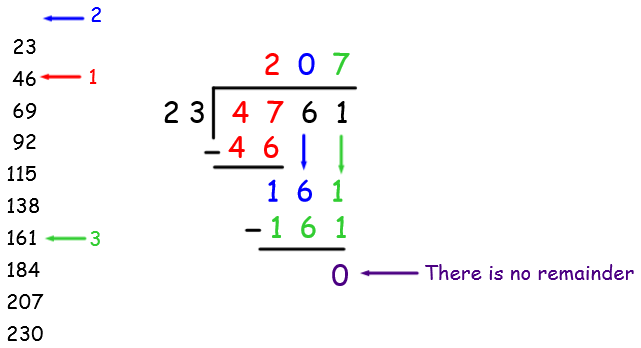d) d) 78 with a remainder of 10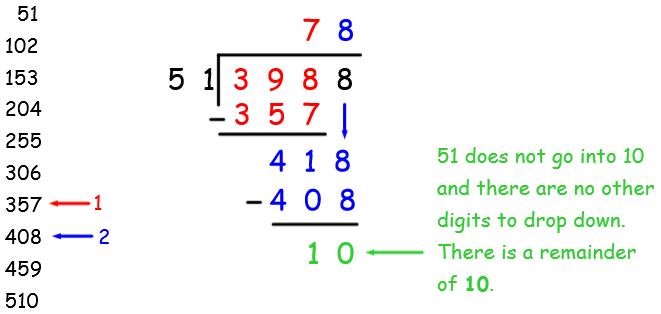### Keep going! Looking for more activities, different subjects or year groups?

Click the button below to view the EdPlace English, maths, science and 11+ activity library